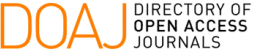### Scaling Symmetries and Conservation Laws for Variable-coefficients Nonlinear Dispersive Equations

Érica M. Silva, Wescley L. Souza

#### Abstract

Scaling symmetries arise in different branches of physics, and symmetry-based approaches are powerful tools for studying scaling-invariant models since they can provide conservation laws that are not obvious by inspection. In this framework, the class of variable-coefficients nonlinear dispersive equations vc\$K(m,n)\$, which contains several important evolution equations modeling nonlinear phenomena, is considered. For some of its scaling-invariant subclasses, we study its nonlinear self-adjointness and construct eight new local conservation laws associated with scaling symmetries by using a general theorem on conservation laws and the multipliers method. The property of scale invariance of those equations led to five conservation laws with a direct physical interpretation: energy, center of mass, and mass are the conserved quantities obtained in some cases.

#### Keywords

Scaling symmetries; Variable-coefficients nonlinear dispersive equations; Nonlinear self-adjointness; Conservation laws

#### Full Text:

PDF

DOI: https://doi.org/10.5540/tema.2019.020.03.429

### Refbacks

• There are currently no refbacks.

Trends in Computational and Applied Mathematics

A publication of the Brazilian Society of Applied and Computational Mathematics (SBMAC)

Indexed in: